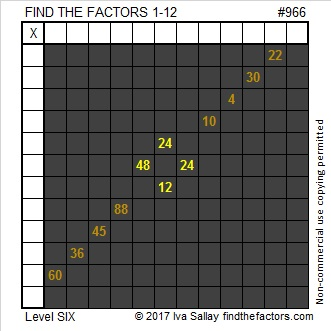# 966 Groan! Gotta Loosen My Belt

If you’ve overeaten this Thanksgiving day, you may be in too much pain to start working off all those extra calories. You may just want to loosen your belt and lie down somewhere while you groan about eating so much. Exercising your brain may help you alleviate some of that regret. This puzzle with its Pilgrim belt buckle could be just what you need. It’s a level 6 so it won’t be easy, but you will feel very accomplished if you can solve it.Print the puzzles or type the solution in this excel file: 12 factors 959-967

Here’s a little information about the number 966:

It is the sum of eight consecutive prime numbers:
103 + 107 + 109 + 113 + 127 + 131 + 137 + 139 = 966

It is also the sum of two consecutive prime numbers:
467 + 479 = 966

966 is palindrome 686 in BASE 12 because 6(144) + 8(12) + 6(1) = 966

• 966 is a composite number.
• Prime factorization: 966 = 2 × 3 × 7 × 23
• The exponents in the prime factorization are 1, 1, 1, and 1. Adding one to each and multiplying we get (1 + 1)(1 + 1)(1 + 1)(1 + 1) = 2 × 2 × 2 × 2 = 16. Therefore 966 has exactly 16 factors.
• Factors of 966: 1, 2, 3, 6, 7, 14, 21, 23, 42, 46, 69, 138, 161, 322, 483, 966
• Factor pairs: 966 = 1 × 966, 2 × 483, 3 × 322, 6 × 161, 7 × 138, 14 × 69, 21 × 46, or 23 × 42
• 966 has no square factors that allow its square root to be simplified. √966 ≈ 31.08054This site uses Akismet to reduce spam. Learn how your comment data is processed.